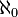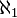# Aleph null facts for kids

Kids Encyclopedia Facts

Aleph null (also aleph naught or aleph 0) is the smallest infinite number. It is the cardinality (size) of the set of natural numbers (there are aleph null natural numbers). Georg Cantor invented and named the concept. The symbol for aleph null is$\aleph_0$.

It is the first infinite number in a series of infinite cardinal numbers. In fact, it is the size of any set which can be matched with the natural numbers. The set of algebraic numbers, for example, has cardinality$\aleph_0$.

Aleph null is followed by aleph one, an infinite number represented by the symbol$\aleph_1$.Aleph null Facts for Kids. Kiddle Encyclopedia.